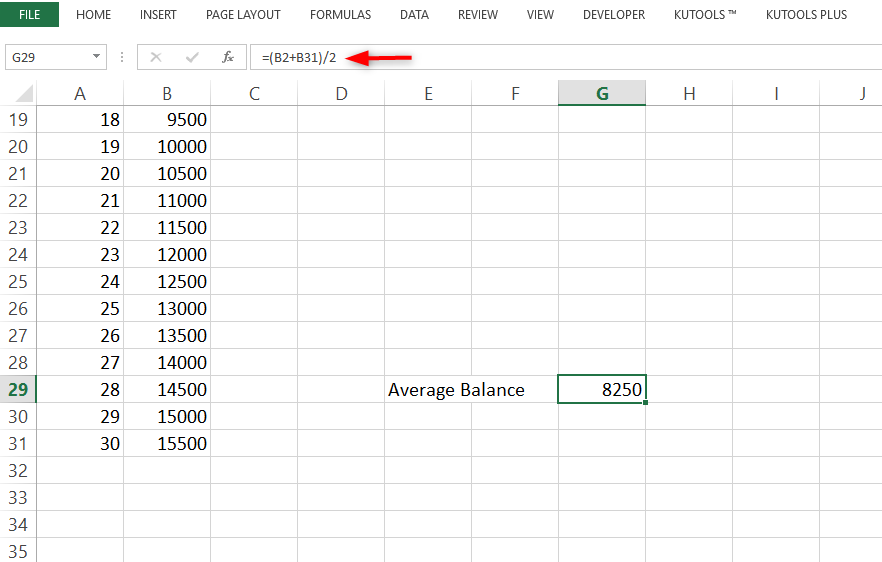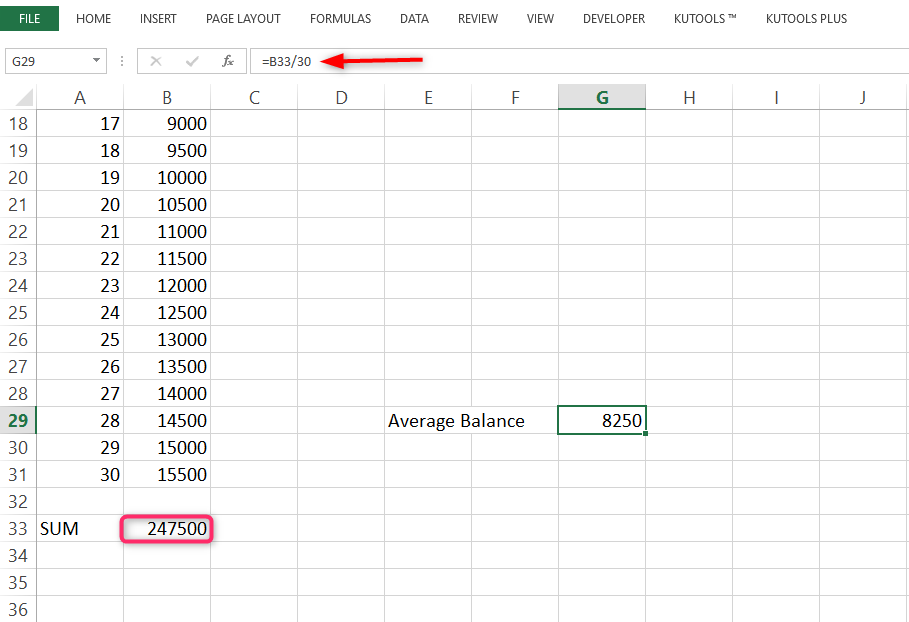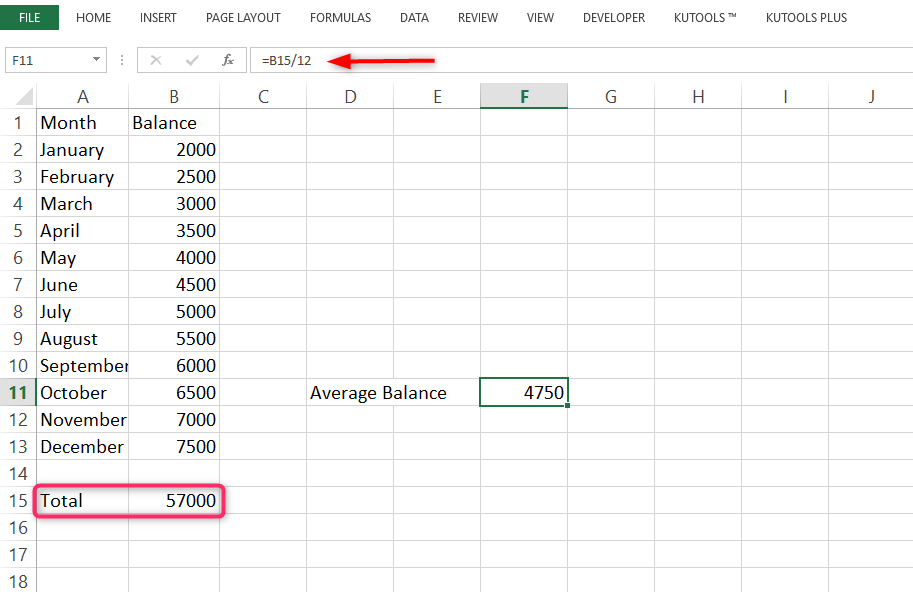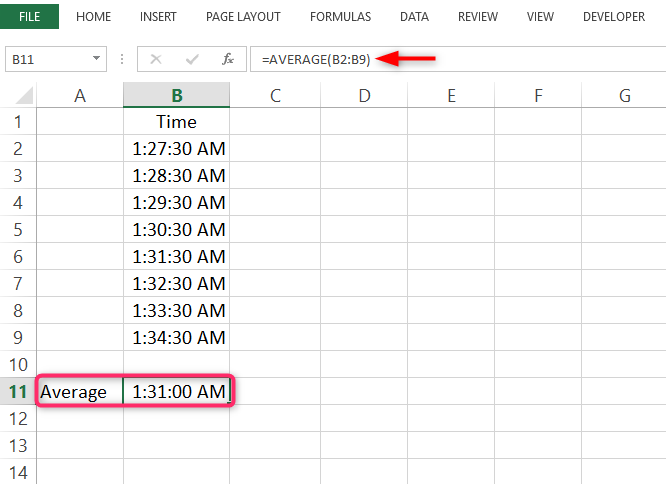# Excel formula for average balance

Average balances are closely associated with bank transactions and credit cards. One can easily calculate his or her average balance depending on the amounts spent.

1. Simple average balance

One can find the average balance by simply taking the initial balance and adding it to the final balance and then dividing the result by two e.g. Average balance at the end of the month = (balance on day1+balance on day 30)/2. To do this in excel:

1. Add a column for balances and a cell for average balance.

2. Type the following formula in the cell

=(B2+B31)/22. Daily average balance

The daily average balance can also be calculated using the sum of all balances as follows:

Total balances in the one-month period/no of days of the month.

To perform this calculation in Excel:

1. Ensure you have a record of balances for each day in the month.

2. Include a cell for average balance. Add all the balances.

3. Type in the following formula to divide the sum of balances by the number of days in the month:

=B33/303. Monthly average balance

Finding the average monthly balance is similar to the daily average balance just that we add total balances and then divide the result by 12.

Excel formula for average Sales

We can find the average sales using the general formula

Average sales= total sales amount/number of periods.

Calculating the average monthly sales.

Average monthly sales =Total sales in year/12 months.

Procedure in Excel.

1. Open Excel.

3. Type the following formula to sum up the sales.

4. Press Enter key.

5. Divide the total by 12.4. Average Daily Sales

ADS= Total sales in a month/number of days.

Calculating average sales per transaction

Average sales can also be calculated depending on the number of transactions conducted

Average sales = Total sales/no of transactions.

Excel formula for average Time

1. Format the times' column to a time format.

2. Also, do the same for the cell into which the average time period is to be placed.

3. Type the following formula into the cell.

=AVERAGE(B2:B9)

4. Press Enter